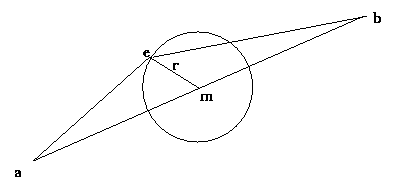# Fjord-Designer

This is a small applet which uses fractal lines to create coastlines.

## Usage:

To use the applet simply draw a line on the applet's canvas.
This line will then be drawn as a (randomly generated) fractal line.

Whenever a new line is drawn, the actual parameters are taken from the input fields.
The values have following meaning:"Div" is the diversion of the line: Given a line with the endpoints `a` and `b`,
the middle point `m` is taken and a new Point `e` is drawn on the circle with radius
`r = div * dist(a,b) * rand`, where `rand` is a random number between 0 and 1.

Then recursively the lines `(a,e)` and `(e,b)` are drawn as fractal lines.
The recursion stops, when `dist(a,b) <= EPS `.

## Buttons

• `Redraw` starts a recalculation of all lines.
• `Clear` deletes all lines.
• `Remove last` removes the last line in the list.

## Remarks:

• Avoid to small ` EPS ` values which cause a stack overflow.
• No typing errors in the input fields are handeled.
• Added 2006-08-09: Over the years I lost the source code, so don't ask for it! :-(